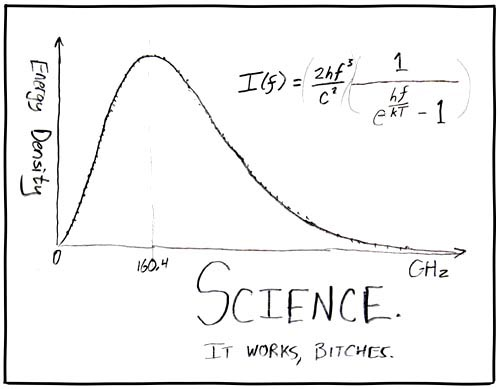Home Newsletter News BooksLanguage/LinguaBooks 3054· Book News · Most clicked · Least clicked

Search for a Book
HELP US TO HELP YOU
Add a new Book## Lectures on Statistical PhysicsLanguage:Author: David TongFormat: Ps, PdfCategory: Statistical MechanicsPages: 194Clicks: 407Description
Contens: 1. Fundamentals of Statistical Mechanics:Introduction; Microcanonical Ensemble; Entropy and the Second Law; Temperature; Two-State Spin System; First Law of Thermodynamics; Canonical Ensemble; Energy Fluctuations; Chemical Potential; Grand Canonical Ensemble. 2. Classical Gases: Classical Partition Functions; Ideal Gas; Equipartition; Maxwell Distribution; Diatomic Gas; Interactions; van der Waals Equation of State; Cluster Expansion; Debye-Huckel model. 3. Quantum Gases:Density of States; Blackbody Radiation; Debye Model of Vibrations in a Solid; Diatomic Gas Revisted, Bose-Einstein Distribution and Bose-Einstein Condensation; Fermi-Dirac Distribution and Fermi Gas; White Dwarfs; Pauli Paramagnetism; Landau Diamagnetism. 4. Classical Thermodynamics: Temperature and the Zeroth Law; The First Law; The Second Law; Carnot Cycles; Entropy; Adiabatic Surfaces; Maxwell Relations; The Third Law. 5. Phase Transitions: Van der Waals equation Revisited; Phase Equilibrium; Maxwell Construction; Clausius-Clapyron Equation; Critical Point; Ising Model; Mean Field Theory; Critical Exponents; Ising Chain; Low Temperature Expansion and Peierls Droplets; High Temperature Expansion; Kramers-Wannier Duality; Landau Theory; Lee-Yang Zeros; Landau-Ginzburg Theory; Fluctuations and Correlations.

Similar Books
 Meccanica statistica Trattatello di Meccanica Statistica Statistical Mechanics. A short treatise Critical Phenomena: An Introduction from a modern perspective Thermal and Statistical Physics Aspects of the ergodic, qualitative,statistical theory of motion Introduction to thermodynamics of spin models Lecture Notes in Statistical Mechanics Statistical mechanics II Statistical Mechanics in a Nutshell Statistical Physics Statistical Mechanics: Entropy, Order Parameters, and Complexity Exactly Solved Models in Statistical mechanics Mecanique Statistique Simulation numérique en physique statistique Thermodynamics & Statistical Mechanics Statistical Mechanics The large deviation approach to statistical mechanics Fluctuation-Dissipation: Response Theory in Statistical Physics A Field Guide to Recent Work on the Foundations of Statistical Mechanics Determination of phase diagrams via computer simulation: Methodology and applications to water, electrolytes and proteins Spin Ice Statistical Physics Notes on the Statistical Mechanics of Systems with Long-Range Interactions On the Statics and Dynamics of Magneto-Anisotropic Nanoparticles Statistical mechanics and dynamics of solvable models with long-range interactions Information Theory and Statistical Physics - Lecture Notes Time-related issues in statistical mechanics Introduction to the field theory of classical and quantum phase transitions Physique statistique des processus irréversibles Statistical mechanics Statistical Physics Statistica Mechanics Percolation Theory A basic introduction to large deviations: Theory, applications, simulations Lecture Notes in Statistical Mechanics and Mesoscopics Statistical Mechanics Statistical Physics of Fracture, Friction and Earthquake Universal scaling relations for logarithmic-correction exponents Statistical Physics Fluctuation-Dissipation: Response Theory in Statistical Physics Statistical Mechanifesto Statistical Physics: a Short Course for Electrical Engineering Students Fluctuation-Dissipation: Response Theory in Statistical Physics Lecture Notes in Thermal Physics Statistical Mechanics Entropy, Order Parameters, and Complexity Modern Statistical Mechanics

```Home |  Authors | About | Contact Us |  Email
Copyright © 2002-2013 FreeScience.info.
Best viewed with Mozilla 1.X 1024x768
```
free scientific books